## domingo, 20 de noviembre de 2016

### Lord's Paradox in R

In an article called A Paradox in the Interpretation of Group Comparisons published in Psychological Bulletin, Lord (1967) made famous the following controversial story:

A university is interested in investigating the effects of the nutritional diet its students consume in the campus restaurant. Various types of data were collected including the weight of each student in the month of January and their weight in the month of June of the same year. The objective of the University is to know if the diet has greater effects on men than on women. This information is analyzed by two statisticians.

The first statistician observes that at the end of the semester (June), the average weight of the men is identical to their average weight at the beginning of the semester (January). This situation also occurs for women. The only difference is that women started the year with a lower average weight (which is obvious from their background). On average, neither men nor women gained or lost weight during the course of the semester. The first statistician concludes that there is no evidence of any significant effect of diet (or any other factor) on student weight. In particular, there is no evidence of any differential effect on both sexes, since no group shows systematic differences.

The second statistician examines the data more carefully. Note that there is a group of men and women who started the semester with the same weight. This group consisted of thin men and overweight women. He notes that those men gained weight from the average and these women lost weight with respect to the average. The second statistician concludes that by controlling for the initial weight, the university diet has a positive differential effect on men relative to women. It is evident that for men and women with the same initial weight, on average they differ since men gained more weight, and women lost more weight.

The following chart shows the reasoning of both statisticians in dealing with the problem. Note that the black line describes a 45 degrees line, the green points are the data coming from the men and the red ones from the women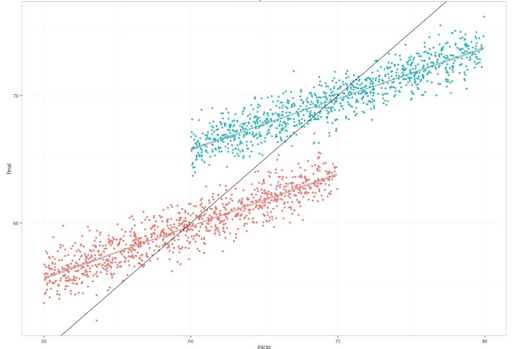The reasoning of the first statistician focuses on the expectations of both distributions. Specifically in the coordinates (x = 60, y = 60), for females, and (x = 70, y = 70) for males, where black, red and green lines appear to coincide. The reasoning of the second statistic is limited to the continuum induced by the overlap of red and green dots. Specifically to the space induced by x = (60, 70), y = (60, 70). Suppose we have access to this dataset as shown in the following illustration, where the first column denotes the initial weight of the students, the second column indicates the final weight, the third column describes the difference between pesos and the last one defines the Sex of the student.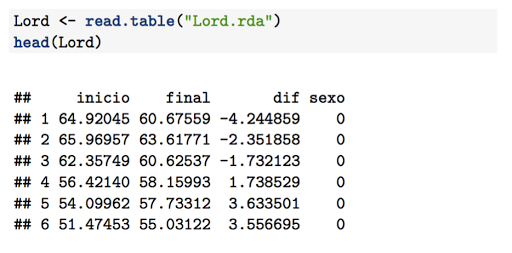The findings of the first statistician are obtained through a simple regression analysis that, taking as a response variable the difference between weights, induces a coefficient of regression equal to zero for the variable sex, which indicates that there are no significant differences in the weight difference between men and women.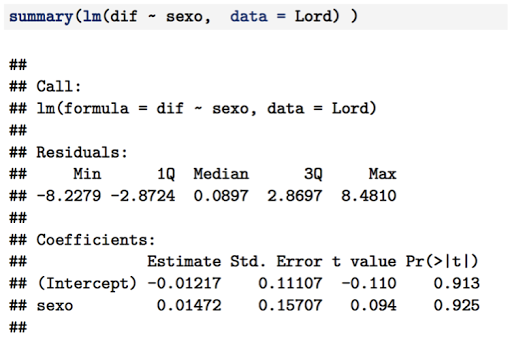The findings of the second statistic are obtained through a covariance analysis, taking as response variable the final weight and covariates are sex and the initial weight of the individual. This method induces a coefficient of regression equal to 5.98 which implies that there is significant difference between the final weight of the people, according to sex.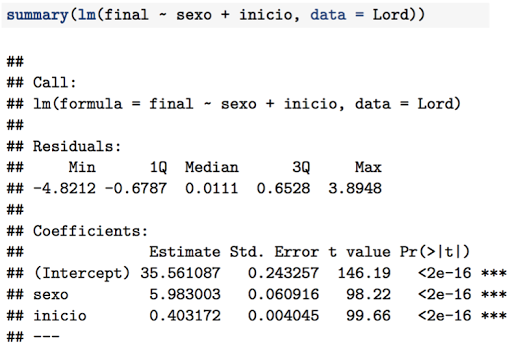For Imbens and Rubin (2015), both are right when it comes to describing the data, although both lack a sound reasoning in establishing some kind of causality between the diet of the university and the loss or gain of weight in the students. Regardless of this I still find more interesting the analysis that arises from the comparison between men and women who started with the same weight (ie all data restricted to x = (60, 70) y = (60, 70).

# R workshop

Lord's paradox summarizes the analysis of two statisticians who analyze the average weight of some students within a particular university. At the end of the semester (June), the average weight of the men is identical to their average weight at the beginning of that six months (January). This situation also occurs for women. The only difference is that women started the year with a lower average weight (which is evident from their natural contexture). On average, neither men nor women gained or lost weight during the semester.

To perform the simulation, we assumed that both the final weight of the men and the women follow a linear relationship with the original weight. Thus, it is assumed that $y_{2i}^M = \beta_0^M + \beta_1 y_{1i}^M + \varepsilon_i$ for the weight of women; and $y_{2i}^H = \beta_0^H + \beta_1 y_{1i}^H + \varepsilon_i$, for the weight of men. Where $y_{1i}^M$ denotes the weight of the $i$-th female at the beginning of the semester, and $y_{2i}^M$ denotes the weight of the $i$-th female at the end of the semester. The notation for men (H) maintains this logic.

Now, note that from their natural contexture, men must have greater weight than women. Suppose that on average the weight of men is equal to that of women plus a constant $c$. In addition, the mean weight in both groups is identical in both times. Then, we have $\bar{y}^M = \beta_0^M + \beta_1 \bar{y}^M$ and that $\bar{y}^H = \beta_0^H + \beta_1 \bar{y}^H = \beta_0^H + \beta_1 (\bar{y}^M + c).$ Hence, after some algebra, we have that $\beta_0^M = (1 - \beta_1) \bar{y}^M$ and $\beta_0^H = \bar{y}^H - \beta_1 (\bar{y}^M + c)$.

The following code replicates a set of data that follows the relationship proposed by Lord.

N <- 1000

b <- 10
l <- 50
u <- 70
Mujer1 <- runif(N, l, u)
Hombre1 <- Mujer1 + b

beta1 <- 0.4
Mujerb0 <- (1 - beta1) * mean(Mujer1)
Hombreb0 <- mean(Hombre1) - beta1 * (mean(Mujer1) + b)

sds <- 1
Mujer2 <- Mujerb0 + beta1 * Mujer1 + rnorm(N, sd=sds)
Hombre2 <- Hombreb0 + beta1 * Hombre1 + rnorm(N, sd=sds)

The graph can be done with the following piece of code:

datos <- data.frame(inicio = c(Mujer1, Hombre1), final = c(Mujer2, Hombre2))
datos$dif <- datos$final - datos$inicio datos$sexo = c(rep(0, N), rep(1, N))

library(ggplot2)
ggplot(data = datos, aes(inicio, final, color = factor(sexo))) +
geom_point() + stat_smooth(method = "lm")  +
geom_abline(intercept = 0, slope = 1) +
ggtitle("Paradoja de Lord") + theme_bw()

#### 5 comentarios:

1.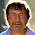Este comentario ha sido eliminado por el autor.

2.Thank you! Interesting and complicated, need to think more. In the meantime, consider rendering the 45° line (identity line) really at 45° by adding "theme(aspect.ratio = 1/1)" to the ggplot chain. (Trivial, I know). Best, Michael

1.Thanks Michael!

3.Este comentario ha sido eliminado por el autor.

4.Hi, I was just thinking about the analysis of the second statistician. If fitting Final = sex + Initial, I believe he is really testing if the intercepts are different which is not the scientific question. So I'm wondering if the analysis instead should be Diff = sex + initial, hence comparing the difference among groups with the same initial weight (therefore controlling for it). Also, I would suggest to do a spaghetti plot (maybe condition on the same weights using colors?).